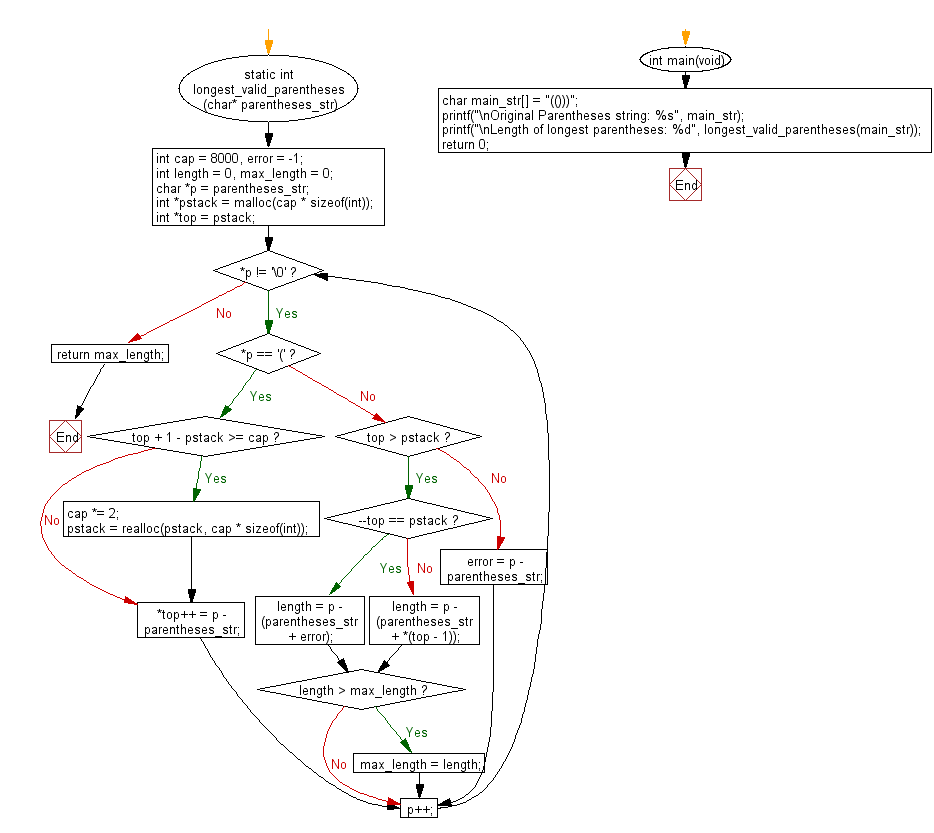﻿ C Program: Length, longest valid parentheses substring# C Exercises: Find the length of the longest valid parentheses substring of a given string

## C Programming Practice: Exercise-16 with Solution

Write a C programming to find the length of the longest valid (correct-formed) parentheses substring of a given string.

C Code:

``````#include <stdio.h>
#include <stdlib.h>

static int longest_valid_parentheses(char* parentheses_str)
{
int cap = 8000, error = -1;
int length = 0, max_length = 0;
char *p = parentheses_str;
int *pstack = malloc(cap * sizeof(int));
int *top = pstack;

while (*p != '\0') {
if (*p == '(') {
if (top + 1 - pstack >= cap) {
cap *= 2;
pstack = realloc(pstack, cap * sizeof(int));
}
*top++ = p - parentheses_str;
} else {
if (top > pstack) {
if (--top == pstack) {
length = p - (parentheses_str + error);
} else {
length = p - (parentheses_str + *(top - 1));
}
if (length > max_length) {
max_length = length;
}
} else {
error = p - parentheses_str;
}
}
p++;
}
return max_length;
}

int main(void)
{
char main_str[] = "(()))";
printf("\nOriginal Parentheses string: %s", main_str);
printf("\nLength of longest parentheses: %d", longest_valid_parentheses(main_str));
return 0;
}
``````

Sample Output:

```Original Parentheses string: (()))
Length of longest parentheses: 4
```

Pictorial Presentation:Flowchart:## C Programming Code Editor:

What is the difficulty level of this exercise?

Test your Programming skills with w3resource's quiz.

﻿

## C Programming: Tips of the Day

What is the difference between printf() and puts() in C?

puts is simpler than printf but be aware that the former automatically appends a newline. If that's not what you want, you can fputs your string to stdout or use printf.

Ref :https://bit.ly/3f2fwcH